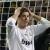## 在 OGRE 中获取对象的方向

```Entity *smith = m_sceneManager->getEntity("Smith");
Bone *head = smith->getSkeleton()->getBone("Bip01 Head");
Vector3 direction = head->_getDerivedOrientation() * Vector3::UNIT_X;
std::cout << StringConverter::toString(direction) << std::endl;```

I thought I should multiply by other than the unit x vector, so I tried all the combinations. In this case (i.e. Smith entity), I got the correct answer using `-Vector3::UNIT_X`, so I thought this is the correct solution. I tried with other entities, but I failed to get the correct answer.

Any idea?

0Multiplying a quaternion by negative Z should correctly return the direction as a vector:

`Vector3 direction = head->_getDerivedOrientation() * Vector3::NEGATIVE_UNIT_Z;`